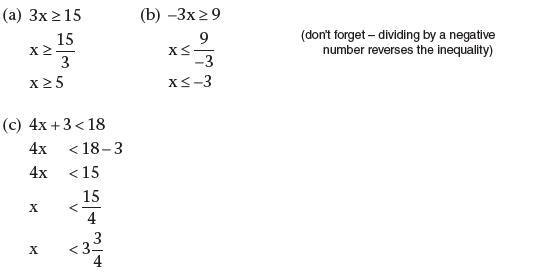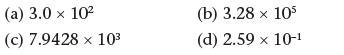# Chapter 3 – Number: fractions, decimals and percentages

Consider the following statements and click to reveal the answer.

1. Reduce these fractions to their simplest forms: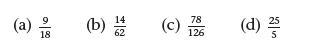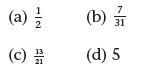2. What are the answers to: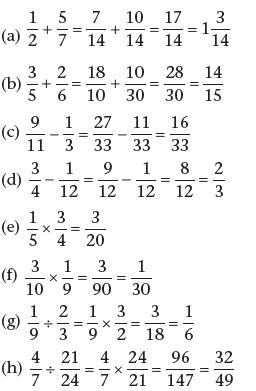3. Convert the following to percentages: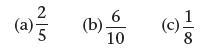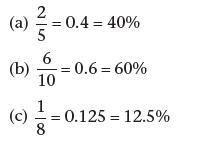4. Calculate the following:

(a) The VAT (at 20%) on £68 (b) 75% of 24 (c) 60% of 80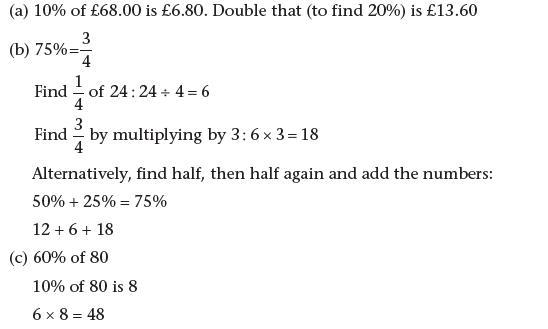5. Solve the following inequalities:

(a) 3x ≥ 15 (b) −3x ≥ 9 (c) 4x + 3 < 18